•#### Sencha Charts Essentials#### Overview of this book

Sencha Charts EssentialsCreditswww.PacktPub.comPrefaceFree Chapter
Fundamentals of Sencha ChartsWorking with Out-of-the-box ChartsSencha Charts ArchitectureCreating a Custom Cartesian ChartCreating a Custom Polar ChartCreating a Custom Spacefilling ChartThemingWorking with Touch GesturesComparison with Other JavaScript Charting LibrariesIndex## Introducing the sample chart

For demonstration and comparison, we will create a chart using Canvas API and SVG, and then we will create the same using Sencha Charts APIs.

Our sample chart, as shown in the following figure, will have:

• x (horizontal) and y (vertical) axes with labels

• A column chart with circular markers in the middle of each bar

• An area chart

• A crosshair that appears on `mousemove`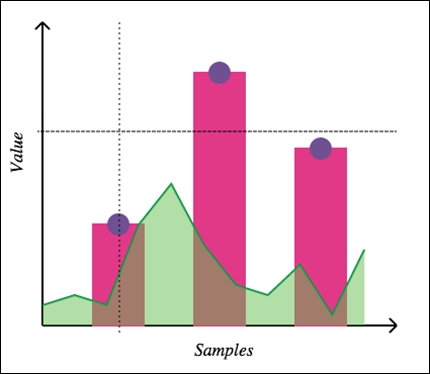In our implementation, we will use a few terms, coordinates, and calculations. They are highlighted in the following diagram: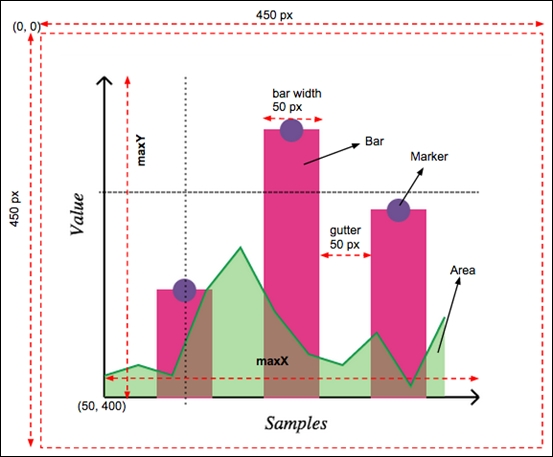### Canvas and SVG

In this section, we will see how we can create the sample chart, described earlier, using Canvas API and SVG. Each of these technologies has their own advantages and context in which they will be used. Their discussion is not in the scope of this book. You may refer to the W3 specifications for their detailed documentation.

### Preparation

First of all, let's prepare our HTML to draw the chart. The Canvas APIs work with the `<canvas>` element, whereas SVG APIs work with the `<svg>` element.

The following table shows the HTML page for Canvas-based and SVG-based drawing approaches. The `ch01_01.html` code, which is on the left-hand side, contains the implementation using Canvas APIs, whereas `ch01_02.html` contains the implementation based on SVG APIs.

`ch01_01.html`

`ch01_02.html`

```<!DOCTYPE HTML>
<html>
<body>
<canvas id="my-canvas" width="450" height="450" style="position: absolute; left: 0; top: 0; z-index: 1;"></canvas>
<canvas id="overlay" width="450" height="450" style="position: absolute; left: 0; top: 0; z-index: 2;"></canvas>

<script>
//code will go here
</script>
</body>
</html>```
```<!DOCTYPE html>
<html>
<body>
<svg id="my-drawing" width="450" height="450" xmlns="http://www.w3.org/2000/svg"

<script>
//code will go here
</script>
</body>

</html>```

All the JavaScript code that we will be writing will go inside the `<script>` tag.

Let's start by creating some helper methods for our chart, which we will use later to create the actual chart. We will have the Canvas and SVG code side by side for easier comparison.

### Tip

You can download the example code files for all Packt books you have purchased from your account at http://www.packtpub.com. If you purchased this book elsewhere, you can visit http://www.packtpub.com/support and register to have the files e-mailed directly to you.

#### Creating a line

To create a line, Canvas provides path APIs—`moveTo` and `lineTo`—whereas SVG provides the `<line>` element. The following screenshot shows the code to create a line, which we will use to create the chart axis, and crosshair lines:

`ch01_01.html`

`ch01_02.html`

```<script>
function createLine(ctx, x1, y1, x2, y2, sw) {
ctx.beginPath();
ctx.moveTo(x1, y1);
ctx.lineTo(x2, y2);
ctx.lineWidth = sw ? sw : 2;
ctx.strokeStyle = 'black';
ctx.stroke();
ctx.closePath();
}```
```<script>
var NS = "http://www.w3.org/2000/svg";
function createLine(x1, y1, x2, y2, sw) {
var line = document.createElementNS(NS, 'line');
line.setAttribute('x1', x1);
line.setAttribute('y1', y1);
line.setAttribute('x2', x2);
line.setAttribute('y2', y2);
line.style.stroke = 'black';
line.style["stroke-width"] = sw ? sw : 2;

return line;
}```

#### Creating an axis

An axis is a combination of a line and an arrow head. In the following code, the `createAxis` method can create an axis and the arrow head based on the line coordinates and the axis `direction` value, which will be `"v"` to indicate vertical axis; or `"h"` to indicate horizontal axis. The `direction` value is used to calculate the path for drawing the arrow head.

In the following table, the code in the first column shows the implementation using the Canvas API, whereas the code in the second column shows the SVG equivalent of it:

`ch01_01.html`

`ch01_02.html`

```  function createAxis(ctx, x1, y1, x2, y2, direction) {
createLine(ctx, x1, y1, x2, y2);

if (direction === "v") {
ctx.beginPath();
ctx.moveTo(x1, y2);
ctx.lineTo(x1 - 10*Math.sin(Math.PI/4), y2 + 10*Math.cos(Math.PI/4));
ctx.moveTo(x1, y2);
ctx.lineTo(x1 + 10*Math.sin(Math.PI/4), y2 + 10*Math.cos(Math.PI/4));
ctx.lineWidth = 2,
ctx.strokeStyle = 'black',
ctx.stroke();
ctx.closePath();
}

if (direction === "h") {
ctx.beginPath();
ctx.moveTo(x2, y1);
ctx.lineTo(x2 - 10*Math.cos(Math.PI/4), x2 - 10*Math.sin(Math.PI/4));
ctx.moveTo(x2, y1);
ctx.lineTo(x2 - 10*Math.cos(Math.PI/4), x2 + 10*Math.sin(Math.PI/4));
ctx.lineWidth = 2;
ctx.strokeStyle = 'black';
ctx.stroke();
ctx.closePath();
}
}```

```  function createPath(p, stroke, fill) {
var path = document.createElementNS(NS, 'path');

path.setAttribute('d', p);
path.style.stroke = stroke ? stroke : 'black';
path.style["stroke-width"] = 2;
path.style.fill = fill ? fill : 'none'

return path;
}

function createAxis(x1, y1, x2, y2, direction) {
var axis = document.createElementNS(NS, 'g');

var line = createLine(x1, y1, x2, y2);
var ah;

if (direction === "v") {
var p = 'M' + x1 + ',' + y2 + ' L' + (x1 - 10*Math.sin(Math.PI/4)) + ',' + (maxY + 10*Math.cos(Math.PI/4));
p += ' M' + x1 + ',' + y2 + ' L' + (x1 + 10*Math.sin(Math.PI/4)) + ',' + (maxY + 10*Math.cos(Math.PI/4));
ah = createPath(p);
}

if (direction === "h") {
var p = 'M' + x2 + ',' + y1 + ' L' + (x2 - 10*Math.cos(Math.PI/4)) + ',' + (x2 - 10*Math.sin(Math.PI/4));
p += ' M' + x2 + ',' + y1 + ' L' + (x2 - 10*Math.cos(Math.PI/4)) + ',' + (x2 + 10*Math.sin(Math.PI/4));
ah = createPath(p);
}

axis.appendChild(line);
axis.appendChild(ah);

return axis;
}```

#### Creating an axis label

The `createLabel` method creates a text label for an axis based on the specified direction. The Canvas approach uses transformation—`translate` and `rotate`—to render the vertical label, whereas the SVG approach uses the `writing-mode style` attribute of the `<text>` element. You may also use `<tspan>` with transformations to show the vertical axis label.

In the following table, the code in the first column shows the implementation of the method using the Canvas API, whereas the code in the second column shows the equivalent implementation using SVG specification:

`ch01_01.html`

`ch01_02.html`

```  function createLabel(ctx, x, y, txt, direction) {

ctx.font = 'Italic 1.1em Aerial';

if (direction === 'v') {
ctx.translate(x, y);
ctx.rotate(-Math.PI/2);
ctx.fillText(txt, 0, 0);

//reset transformation
ctx.setTransform(1, 0, 0, 1, 0, 0);
} else {
ctx.fillText(txt, x, y);
}
}```
```  function createLabel(x, y, txt, direction) {
var text = document.createElementNS(NS, 'text');

text.setAttribute('x', x);
text.setAttribute('y', y);
text.style.font = 'Italic 1.1em Aerial';
if (direction === 'v') {
text.style['writing-mode'] = 'tb';
}

var node = document.createTextNode(txt);
text.appendChild(node);

return text;
}```

#### Creating a bar

To create a bar for our column chart, the `createBar` method draws a rectangle using the `rect` API of Canvas and the `<rect>` element of SVG.

`ch01_01.html`

`ch01_02.html`

```  function createBar(ctx, x, y, w, h) {
ctx.beginPath();
ctx.moveTo(x, y);
ctx.rect(x, y, w, h);

ctx.fillStyle = '#E13987';
ctx.lineWidth = 2;
ctx.strokeStyle = '#E13987';
ctx.stroke();
ctx.fill();
ctx.closePath();
}```

```  function createBar(x, y, w, h) {
var rect = document.createElementNS(NS, 'rect');

rect.setAttribute('x', x);
rect.setAttribute('y', y);
rect.setAttribute('width', w);
rect.setAttribute('height', h);
rect.style.fill = '#E13987';
rect.style.stroke = '#E13987';
rect.style['stroke-width'] = 2;

return rect;
}```

#### Creating a marker on the bar

In our chart, we want to show circular markers on the top of the bars. The `createMarker` method will help us add markers to our drawing based on the location of its center and radius.

`ch01_01.html`

`ch01_02.html`

```  function createMarker(ctx, cx, cy, r) {
ctx.beginPath();

ctx.arc(cx, cy, r, 0, 2*Math.PI, false);
ctx.fillStyle = '#6F5092';
ctx.lineWidth = 2;
ctx.strokeStyle = '#6F5092';
ctx.stroke();
ctx.fill();

ctx.closePath();
}```

```  function createMarker(cx, cy, r) {
var circle = document.createElementNS(NS, 'circle');

circle.setAttribute('cx', cx);
circle.setAttribute('cy', cy);
circle.setAttribute('r', r);
circle.style.fill = '#6F5092';
circle.style.stroke = '#6F5092';
circle.style['stroke-width'] = 2;

return circle;
}```

This ends the list of helper methods that we need to create our chart. Let's see how we can use them to create the final output.

### Creating a chart

Now, we will enhance our script to create the chart in a step-by-step approach. We will start with the axis.

#### Axes

Before we start, we first need access to our drawing surface, either the `canvas` or `<svg>` element. Since we have already set the ID on the element, we can use the `document.getElementById` method to access them. Canvas API, however, requires us to additionally get the drawing context and use it for the drawing.

Once we have access to the drawing surface, we use the `createAxis` method to create the x and y axes, as shown in the following table:

`ch01_01.html`

`ch01_02.html`

```  var canvas = document.getElementById('my-canvas');
var ctx = canvas.getContext('2d');

var samples = [100, 250, 175],
gutter = 50,
barWidth = 50,
x0 = 50,
y0 = 400,

//draw axes
var maxX = x0 + samples.length*(barWidth + gutter) + gutter;
var maxY = y0 - 250 - 50;
createAxis(ctx, x0, y0, maxX, y0, 'h');
createAxis(ctx, x0, y0, x0, maxY, 'v');```

```  var svg = document.getElementById('my-drawing');

var samples = [100, 250, 175],
gutter = 50,
barWidth = 50,
x0 = 50,
y0 = 400,

//draw axes
var maxX = x0 + samples.length*(barWidth + gutter) + gutter;
var maxY = y0 - 250 - 50;
var xAxis = createAxis(x0, y0, maxX, y0, 'h');
var yAxis = createAxis(x0, y0, x0, maxY, 'v');

svg.appendChild(xAxis);
svg.appendChild(yAxis);```

The following screenshot shows the output with x and y axes. Each axis has an arrowhead as well: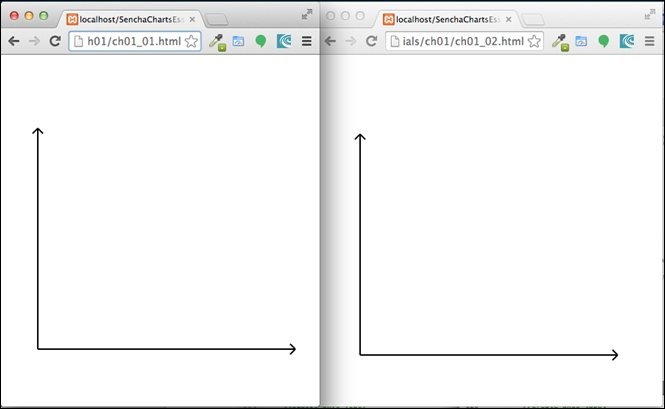#### Axis label

To draw a label on an axis, use the `createLabel` method, as shown in the following table:

`ch01_01.html`

`ch01_02.html`

```  //create axis label
createLabel(ctx, maxX/2, y0 + 30, 'Samples');
createLabel(ctx, x0 - 20, y0 - (y0 - maxY)/2, 'Value', 'v');```

```  //create axis label
var xLabel = createLabel(maxX/2, y0 + 30, 'Samples');
var yLabel = createLabel(x0 - 20, y0 - (y0 - maxY)/2, 'Value', 'v');

svg.appendChild(xLabel);
svg.appendChild(yLabel);```

The following output is produced to show the axis label: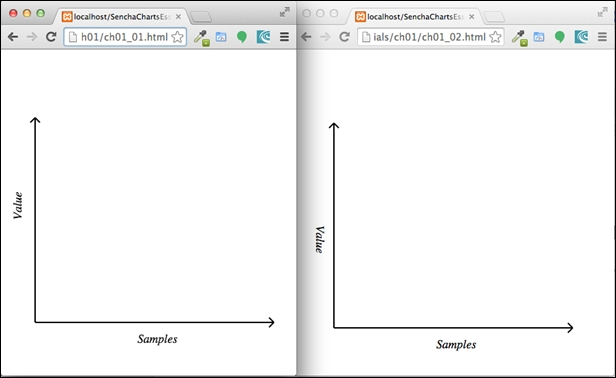#### Bar chart with a marker

To create the bar for each sample data, we will use the `createBar` method and `createMarker` to show the marker on the top of each bar, as shown in the following table:

`ch01_01.html`

`ch01_02.html`

```  //draw bars
for (var i=0; i<samples.length; i++) {
var x, y, w = barWidth, h = samples[i];
x = x0 + gutter + i*(w + gutter);
y = y0 - h;

createBar(ctx, x, y, w, h);
createMarker(ctx, x + w/2, y, markerRadius);
}```

```  //draw bars
for (var i=0; i<samples.length; i++) {
var x, y, w = barWidth, h = samples[i];
x = x0 + gutter + i*(w + gutter);
y = y0 - h;

var bar = createBar(x, y, w, h);
var marker = createMarker(x + w/2, y, markerRadius);

svg.appendChild(bar);
svg.appendChild(marker);

}```

You may refer to the second figure in the Introducing the sample chart section for the `barWidth` and `gutter` values. The following screenshot shows the output produced from the two applications: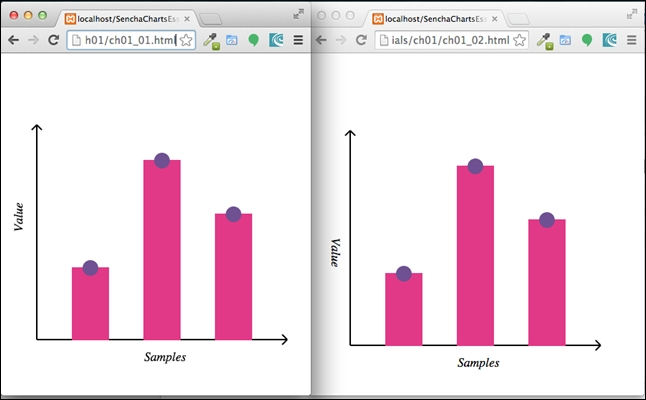#### Creating an area chart with line stroking

Our next step is to create an area chart. First, we will show the line and then we will fill the area.

The following code shows how to create the area chart with line stroke using a different sample—`areaSamples`:

`ch01_01.html`

`ch01_02.html`

```  //draw area chart
var areaSamples = [20, 30, 20, 100, 140, 80, 40, 30, 60, 10, 75];
var n = areaSamples.length;
var d = (maxX - x0)/n;
//distance between the points
var start = true;
for (var i=0; i<n; i++) {
var x = x0 + i*d , y = y0 - areaSamples[i];

if (start) {
ctx.beginPath();
ctx.moveTo(x, y);
start = false;
}
ctx.lineTo(x, y);
}
ctx.lineWidth = 2,
ctx.strokeStyle = '#00904B',
ctx.stroke();
ctx.closePath();```

```  //draw area chart
var areaSamples = [20, 30, 20, 100, 140, 80, 40, 30, 60, 10, 75];
var n = areaSamples.length;
var d = (maxX - x0)/n;
//distance between the points
var start = true;
var p = '';
for (var i=0; i<n; i++) {
var x = x0 + i*d , y = y0 - areaSamples[i];

if (start) {
p += 'M' + x + ',' + y;
start = false;
}

p += ' L' + x + ',' + y;
}

//area - with border
var area = createPath(p, '#00904B');
svg.appendChild(area);```

We have used path APIs to create the line chart, which we will fill in the next section to make it an area chart. Run the two codes and you will see the following output: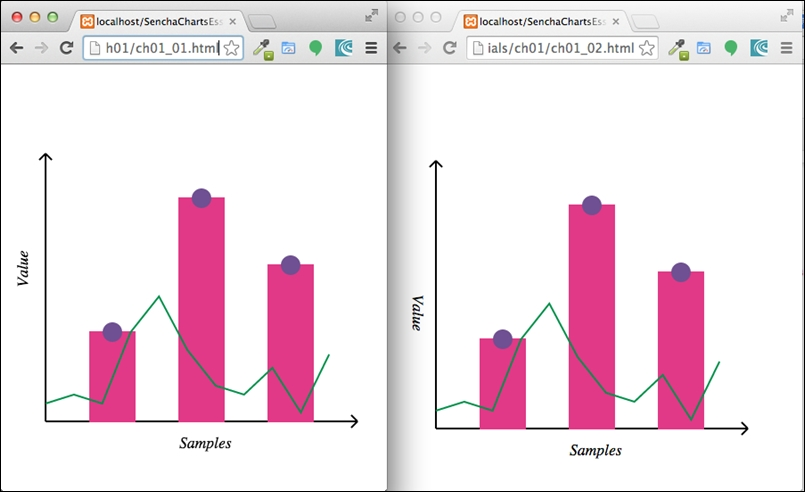#### Creating an area chart with fill

Now, let's fill the area under the line chart that we drew earlier to make it an area chart. To fill the area, we will have to end the path at its starting point.

Since we have added the area chart after the bar, the bar would be hidden behind the area chart. So, we will set the transparency when we fill the area. This way, the user can see the bar behind the area. To set the transparency in Canvas, we set the `globalAlpha` parameter, whereas in SVG, it is a bit more involved. We have to create a `mask` element with transparency level and use it to fill the area.

In the following table, the code in the first column shows the implementation using the Canvas API, whereas, the code in the second column shows the corresponding implementation using SVG:

`ch01_01.html`

`ch01_02.html`

```  //fill the area chart
start = true;
ctx.globalAlpha = 0.5;
ctx.fillStyle = '#64BD4F';
for (var i=0; i<n; i++) {
var x = x0 + i*d , y = y0 - areaSamples[i];

if (start) {
ctx.beginPath();
ctx.moveTo(x, y0);
start = false;
}
ctx.lineTo(x, y);

if (i === (n - 1)) {
ctx.lineTo(x, y0);
}
}
ctx.fill();
ctx.closePath();```

```  //fill the area chart
p += ' L' + x + ',' + y0 + ' L' + x0 + ',' + y0 + ' Z';
var fillArea = createPath(p, 'none', '#64BD4F');

//transparency for the fill area
var defs = document.createElementNS(NS, 'defs');
var fillArea1 = document.createElementNS(NS, 'path');
fillArea1.setAttribute('d', p);
fillArea1.style.fill = '#666666';
svg.appendChild(defs);

fillArea.style.fill = '#64BD4F';
svg.appendChild(fillArea);```

The following screenshot shows the output produced by these two codes: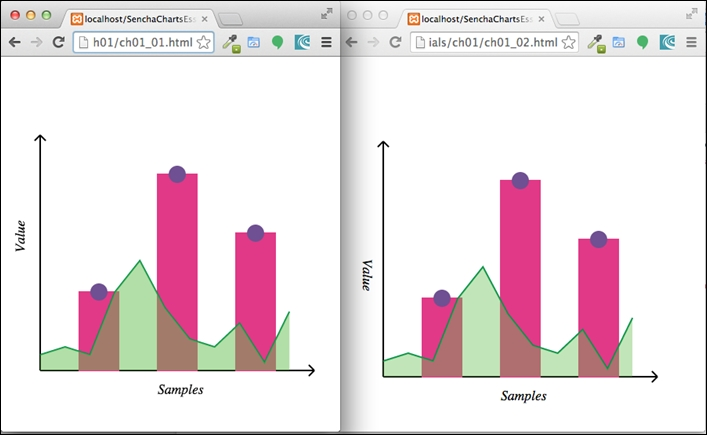#### Crosshair lines

The last item we are left with is crosshair lines. To show the crosshair line on `mousemove` in the Canvas approach, we will add one more `<canvas>` element on top (that is, higher `z-index`) of the existing one as an overlay layer. It is this `overlay` canvas that we will use to render crosshair lines. Here is the code snippet showing an additional overlay `<canvas>` element added to the `document` body:

```<body>
<canvas id="my-canvas" width="450" height="450" style="position: absolute; left: 0; top: 0; z-index: 1;"></canvas>
<canvas id="overlay" width="450" height="450" style="position: absolute; left: 0; top: 0; z-index: 2;"></canvas>
<script>
...```

We register the handler for `mousemove` on the `overlay` canvas. The handler clears the canvas using the `clearRect` API so that old lines get cleared before adding the crosshair lines.

In the SVG approach, we will use the same `<svg>` element. The lines are added beforehand and the handler code moves them to the new location based on mouse position by setting their coordinate related attributes.

There is a check we can make to ensure that we don't show the crosshair if the mouse is outside of the chart area.

The following table shows the code related to crosshair for Canvas as well as SVG:

`ch01_01.html`

`ch01_02.html`

```  //show cross-hair
var overlay = document.getElementById('overlay');
var overCtx = overlay.getContext('2d');

var lineDash = overCtx.getLineDash();
overCtx.setLineDash([5,5]);
overCtx.clearRect(0, 0, overlay.width, overlay.height);
var rect = overlay.getBoundingClientRect();
var x = evt.clientX - rect.left,
y = evt.clientY - rect.top;
//don't show the cross-hair if we are outside the chart area
if (x < x0 || x > maxX || y < maxY || y > y0) {
return;
}

overCtx.beginPath();
overCtx.moveTo(x0 - 5, y);
overCtx.lineTo(maxX, y);
overCtx.moveTo(x, maxY);
overCtx.lineTo(x, y0 + 10);
overCtx.strokeStyle = 'black',
overCtx.stroke();
overCtx.closePath();
}, false);```

```  //show cross-hair
var hl = createLine(-x0, -y0, -maxX, -y0);
var vl = createLine(-x0, -y0, -x0, -maxY);
hl.style['stroke-dasharray'] = [5,5];
vl.style['stroke-dasharray'] = [5,5];
svg.appendChild(hl);
svg.appendChild(vl);
var x = evt.offsetX || evt.clientX,
y = evt.offsetY || evt.clientY;
//don't show the cross-hair if we are outside the chart area
if (x < x0 || x > maxX || y < maxY || y > y0) {
return;
}

hl.setAttribute('x1', x0 - 5);
hl.setAttribute('y1', y);
hl.setAttribute('x2', maxX);
hl.setAttribute('y2', y);

vl.setAttribute('x1', x);
vl.setAttribute('y1', maxY);
vl.setAttribute('x2', x);
vl.setAttribute('y2', y0 + 10);

});```

Here is the output of the two codes showing the crosshair lines when a user moves the mouse in the chart area: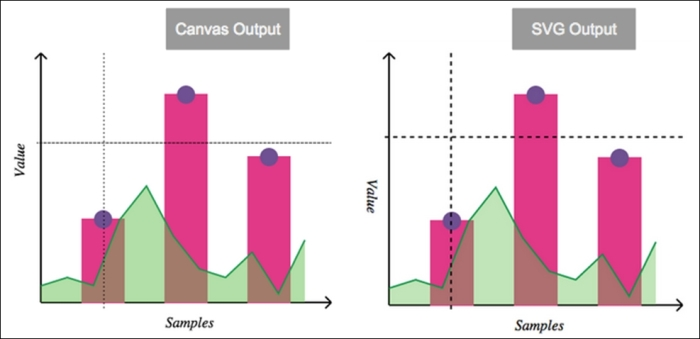So, we have our final output. Great! But wait! Imagine that you are a charts library developer or someone who wants to add charting capability to your application and has to support different browsers; some of them support SVG (for example, older ones), whereas some of them support Canvas. You can visit http://caniuse.com/ to review specific browser-related support. To ensure that your charts render on both types of browser, you will have to implement your code in SVG as well as Canvas, and then, based on the browser support, your code has to use one of them. The problem becomes even bigger if you have to support old IE browsers that support VML, as the APIs and approaches differ.

This is exactly the kind of problem we can solve using an abstraction called Surface, which is offered by Sencha Charts. Let's look at the Surface abstraction, what mechanism it offers for drawing, and how we can use them to create the same chart that we have created using the SVG and Canvas APIs.